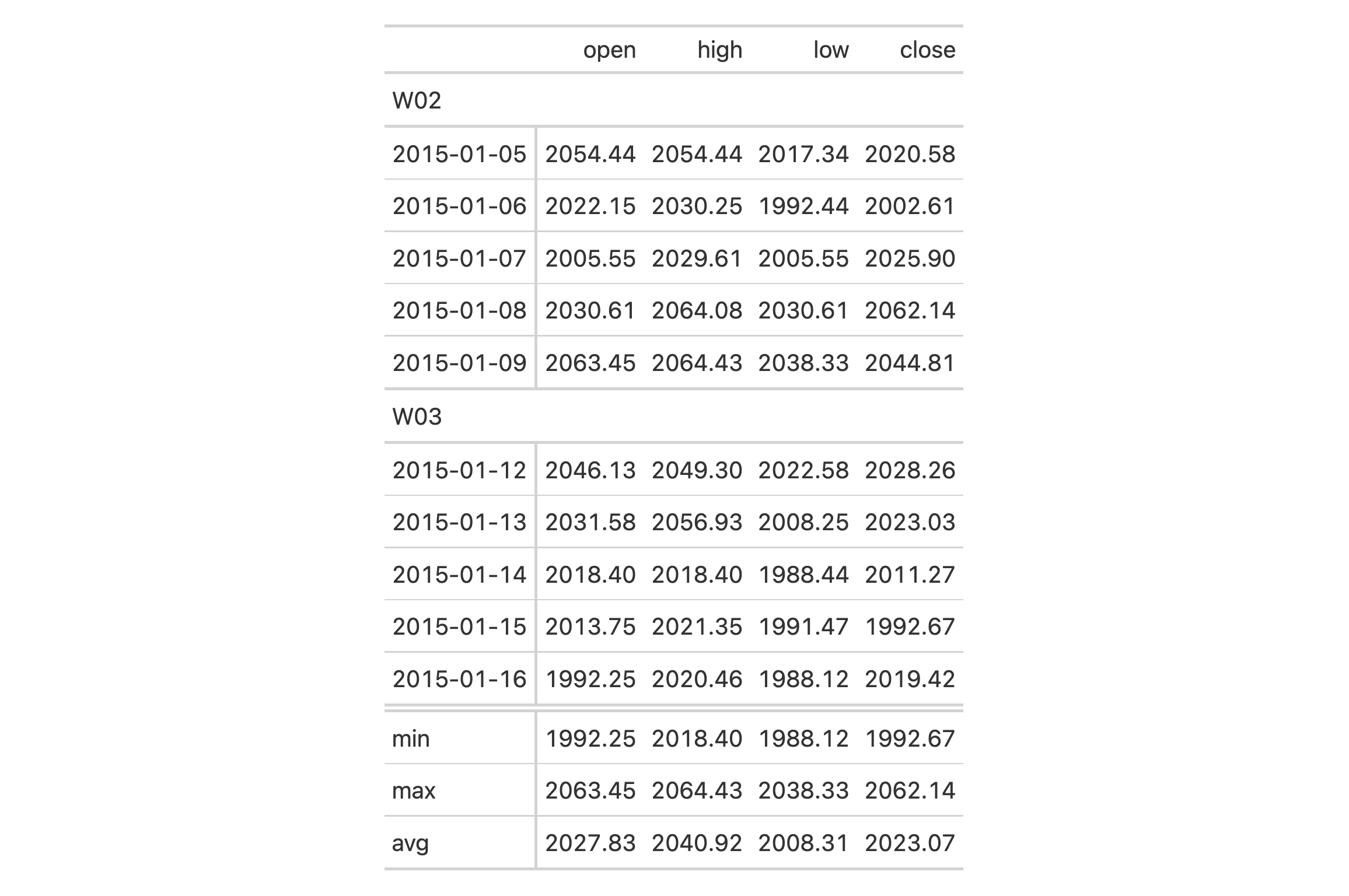Add grand summary rows to the gt table by using applying aggregation functions to the table data. The summary rows incorporate all of the available data, regardless of whether some of the data are part of row groups. You choose how to format the values in the resulting summary cells by use of a formatter function (e.g, fmt_number) and any relevant options.

## Usage

grand_summary_rows(
data,
columns = everything(),
fns,
missing_text = "---",
formatter = fmt_number,
...
)

## Arguments

data

A table object that is created using the gt() function.

columns

The columns for which the summaries should be calculated.

fns

Functions used for aggregations. This can include base functions like mean, min, max, median, sd, or sum or any other user-defined aggregation function. The function(s) should be supplied within a list(). Within that list, we can specify the functions by use of function names in quotes (e.g., "sum"), as bare functions (e.g., sum), or as one-sided R formulas using a leading ~. In the formula representation, a . serves as the data to be summarized (e.g., sum(., na.rm = TRUE)). The use of named arguments is recommended as the names will serve as summary row labels for the corresponding summary rows data (the labels can derived from the function names but only when not providing bare function names).

missing_text

The text to be used in place of NA values in summary cells with no data outputs.

formatter

A formatter function name. These can be any of the fmt_*() functions available in the package (e.g., fmt_number(), fmt_percent(), etc.), or a custom function using fmt(). The default function is fmt_number() and its options can be accessed through ....

...

Values passed to the formatter function, where the provided values are to be in the form of named vectors. For example, when using the default formatter function, fmt_number(), options such as decimals, use_seps, and locale can be used.

## Value

An object of class gt_tbl.

## Details

Should we need to obtain the summary data for external purposes, the extract_summary() function can be used with a gt_tbl object where grand summary rows were added via grand_summary_rows().

## Examples

Use sp500 to create a gt table with row groups. Create the grand summary rows min, max, and avg for the table with the grand_summary_rows() function.

sp500 %>%
dplyr::filter(date >= "2015-01-05" & date <= "2015-01-16") %>%
dplyr::arrange(date) %>%
dplyr::mutate(week = paste0("W", strftime(date, format = "%V"))) %>%
gt(
rowname_col = "date",
groupname_col = "week"
) %>%
grand_summary_rows(
columns = c(open, high, low, close),
fns = list(
min = ~min(.),
max = ~max(.),
avg = ~mean(.)),
formatter = fmt_number,
use_seps = FALSE
)## Function ID

5-2

Other row addition/modification functions: row_group_order(), summary_rows()